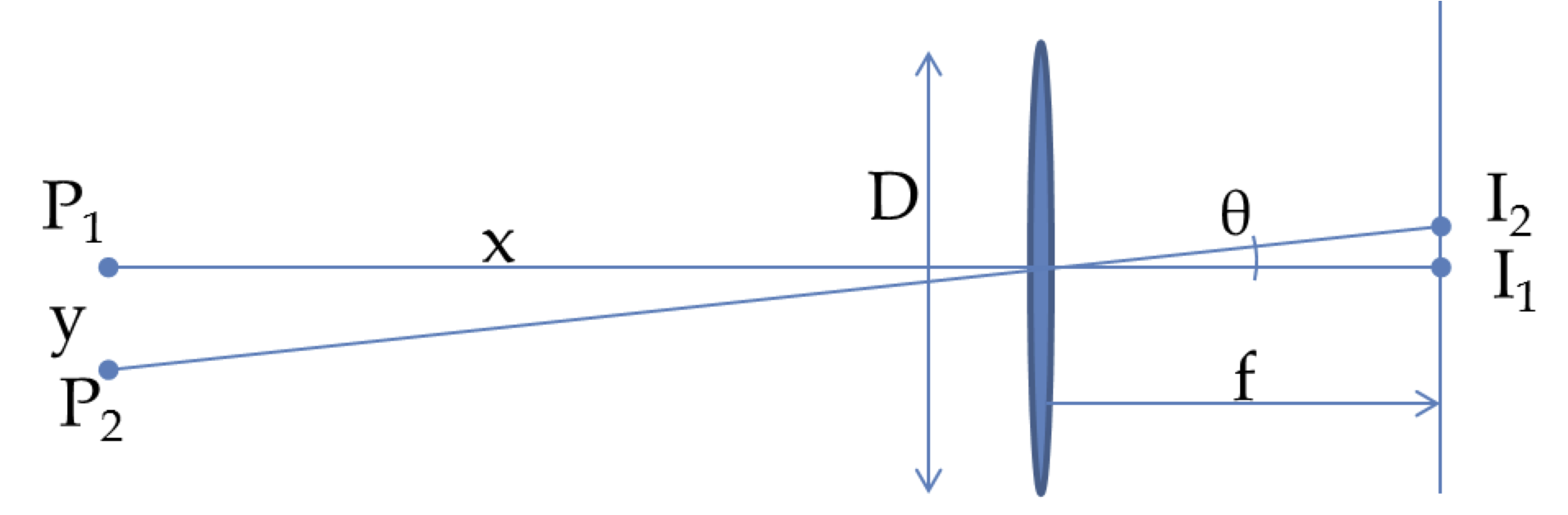## Section51.9Diffraction of Light Bootcamp

### Subsection51.9.5Miscellaneous

A single slit is illuminated by two light waves of wavelengths 440 nm and an unknown $\lambda$ such that the second diffraction minimum of 440-nm coincides with the third diffraction minimum for $\lambda\text{.}$ Find $\lambda\text{.}$
Hint

Equate the sines of the angles of the two conditions.

$293.3\:\textrm{nm}\text{.}$

Solution

Equating the angles is the same as equating the sines of those angles. Therefore, we will get the following condition from the given data.

\begin{equation*} \dfrac{2\times 440\: \textrm{nm}}{a} = \dfrac{3\:\lambda}{a},\ \ \Longrightarrow\ \ \lambda = 293.3\:\textrm{nm}. \end{equation*}
Prove that in a double-slit experiment if the width $b$ and the center-to-center distance $a$ are related by $a = p b\text{,}$ then the number of peaks inside the central maximum of the diffraction peak is $2p+1\text{.}$
Hint

First find half width or $m^\prime=1$ minimum of the central peak of diffraction, and, then find the maximum interference order for that angle.

$2p+1\text{.}$

Solution

The half width of the central peak of the diffraction pattern gives

\begin{equation*} b\:\sin\theta_{\textrm{hw}} = \lambda. \ \ \ \ (1) \end{equation*}

Within this angle the maximum constructive interference order will be

\begin{equation*} m_{\textrm{max}}\: \lambda = a\:\sin\theta_{\textrm{hw}}.\ \ \ \ (2) \end{equation*}

The total number of constructive interference max will be

\begin{equation*} n = 2 m_{\textrm{max}} + 1\ (\textrm{including } m = 0) = 2 p + 1\ \ (\textrm{using (1) and (2).}) \end{equation*}

Red light (wavelength 632.8 nm in air) from a helium-neon laser is incident on a single slit of width 0.05 mm. The entire apparatus is immersed in water of refractive index $\frac{4}{3}\text{.}$ Determine the angular width of the central peak.

Hint

Wavelength wil lbe different in water.

$0.19\:\textrm{rad}\text{.}$

Solution

The wavelength in water, $\lambda = \lambda_0/n = 474.6$ nm. The angular width will be obtained from twice the angle for $m=1$ diffraction minimum.

\begin{align*} \Delta \theta \amp = 2\theta_1\\ \amp = 2\:\sin^{-1}\left(\dfrac{\lambda}{b} \right)=2\:\sin^{-1}\left(\dfrac{ 474.6\:\textrm{nm}}{0.05\: \textrm{mm}} \right)\\ \amp = 0.19\:\textrm{rad}. \end{align*}

A light ray of wavelength 461.9 nm emerges from a 2 mm circular aperture of a krypton ion laser. Due to diffraction the beam expands as it moves out. How large is the central bright spot at (a) 1 m, (b) 1 km, (c) 1000 km, and (d) at the surface of the Moon at a distance of 400,000 km from the Earth.

Hint

Find angular width in radians first, then multiply it by distance.

(a) $0.28\: \textrm{mm}\text{,}$ (b) $280\: \textrm{mm}\text{,}$ (c) $280\: \textrm{m}\text{,}$ (d) $112\: \textrm{km}\text{.}$

Solution

We start with the angular width calculation. $\Delta \theta = 1.22 \dfrac{\lambda}{D} = 1.22\: \dfrac{ 461.9\:\textrm{nm}}{2\: \textrm{mm}} = 0.00028\:\textrm{rad}.$ Using $L\Delta \theta$ we find the diameter of the beam at different $L$'s.

\begin{align*} \amp \textrm{(a)}\ \ 0.28\: \textrm{mm},\\ \amp \textrm{(b)}\ \ 280\: \textrm{mm},\\ \amp \textrm{(c)}\ \ 280\: \textrm{m},\\ \amp \textrm{(d)}\ \ 112\: \textrm{km}. \end{align*}

A spy satellite is reputed to be able to resolve objects 10 cm apart while operating 200 km above the surface of the Earth. What is the diameter of the aperture of the telescope if the resolution is only limited by the diffraction effects? Use 550 nm for light.

Hint

At the Raleigh criterion, angle of separation will equal width of the Airy's disk.

$1.34\text{ m}\text{.}$

Solution

We can use the same setup as we used for resolving objects on the moon.Here, we want the angular separation, $\theta\approx y/x$ to be greater than the angular width of the Airy's disk, namely, $\theta_R=1.22\lambda/D\text{.}$ Therefore, we get at-the-Raleigh-criterion to be

\begin{equation*} \frac{y}{x} = 1.22\frac{\lambda}{D}. \end{equation*}

Therefore,

\begin{equation*} D = 1.22\frac{ x \lambda}{y} = 1.22\frac{ 200\times 10^3 \times 550\times 10^{-9}}{0.1} = 1.34\text{ m}. \end{equation*}